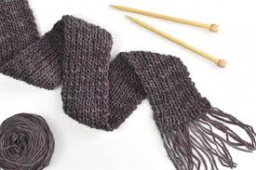# Knitting a scarf

Rebecca is knitting a scarf. She runs out of yarn when the scarf is 1 1/2 feet long, which is ⅔ of the length she wants to make. How long is the scarf when finished? Write a multiplication and division equation to represent this situation.

x =  2 14 = 9/4 ft

### Step-by-step explanation:Did you find an error or inaccuracy? Feel free to write us. Thank you!

Tips for related online calculators
Need help calculating sum, simplifying, or multiplying fractions? Try our fraction calculator.
Need help with mixed numbers? Try our mixed-number calculator.
Do you have a linear equation or system of equations and looking for its solution? Or do you have a quadratic equation?
Do you want to convert length units?